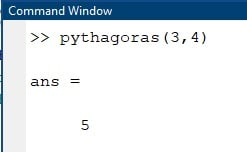Matlab

# Scripts and Functions in Matlab explained with Example Codes

## Scripts and Functions in MATLAB:

scripts and functions in Matlab- In this tutorial, i will be discussing the difference between script and function. Whenever you are going to perform a specific functionality by using MATLAB code you either go for a MATLAB script or a MATLAB function. MATLAB script is the simplest form of the MATLAB file in which you can write your MATLAB code in any way allowed by the MATLAB rules. I am using MATLAB 2021a so to generate a script you go on this option new and click this script.So by clicking the script a blank file will be openedand in this blank file you can write your own code in any format allowed by MATLAB coding rules.Generally, it is a good practice to write a couple of commands at the start of the file which are used to clear all the variables and the command window.The first one is clc which is used for clear screen and the second one is clear this command is used to clear all the variables from the workspace.

Clc

Clear all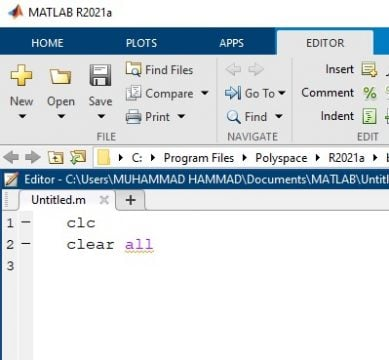Now let’s suppose i want to add three numbers and multiply the same numbers. So first of all i will initialize or declare the three variables and for example these three variables are:

A = 1

B = 2

C = 3

Now the next command is the sum of these three variables so that is sum is equal to:

A + B + C

The next command is the product:

A  * B * C

Now it is a simple code which is adding three numbers and multiplying the same numbers and saving the values in these two variables named as sum and product.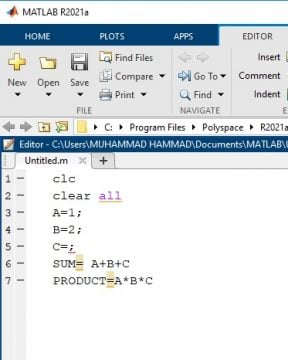Now before running the scriptwe need to save this file so you simply go to the file and click save.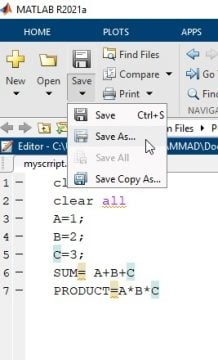Now a dialog box will appear and select any directory where you want to save i am going to the default directory which is MATLAB in the documents folder and one important point is that i can save it by any name of my own choice. So i am writing here myscript.m and click on the save button now this file is saved.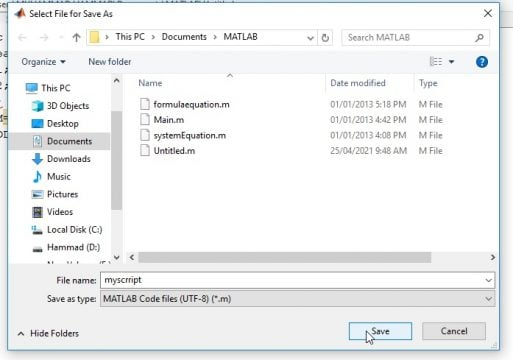Now I want to run the code we will simply click on the RUN or we can also run the program by pressing F5 button.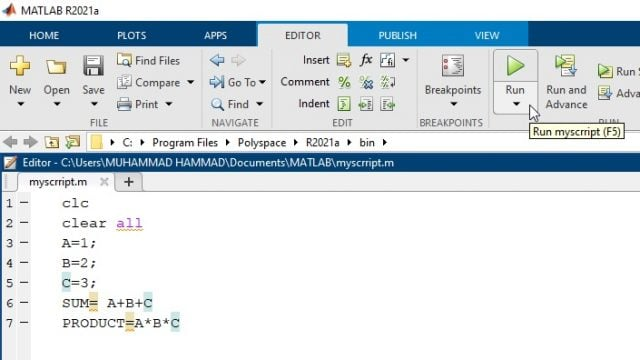So now going to command window you can see that the file is successfully run and the result is shown in the command window.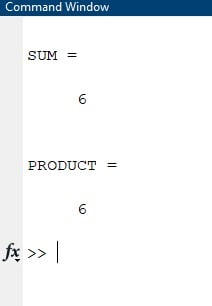Now if i want to generate the same functionality by using MATLAB function.i will simply go to the new option and select function.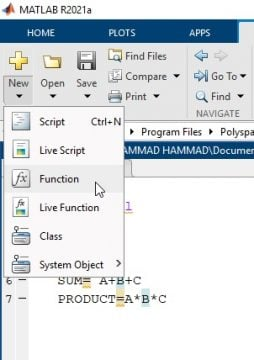You can see that MATLAB has provided a built-in template to write the function so what is the main concept of function.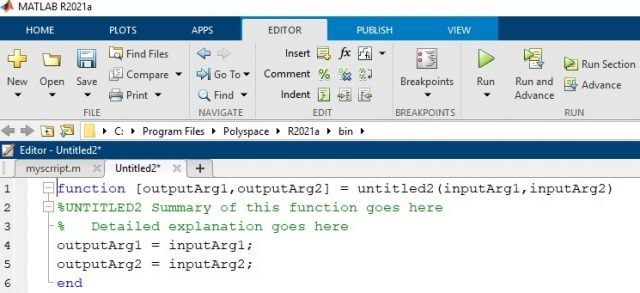It takes multiple or single input arguments to perform a specific functionality and the output is returned in the output variables declared in the syntax of function.The structure of a user-defined function the first line in a function file must begin with a function definition line that contains the functions inputs and outputs the output arguments are the variables whose values are calculated by the function using the provided input arguments the output arguments are enclosed in square brackets though the brackets are optional if there is only one output the input arguments are enclosed with parentheses. The function name is the same name that the file and an end statement are used to terminate a function.

Since we are going to perform SUM and product of three variables. So now in order to proceed for this functionality we will insert these three inputs as the inputarguments and input arguments will be entered in the function and replace the inputArg1,inputArg2 with name a,b ,c.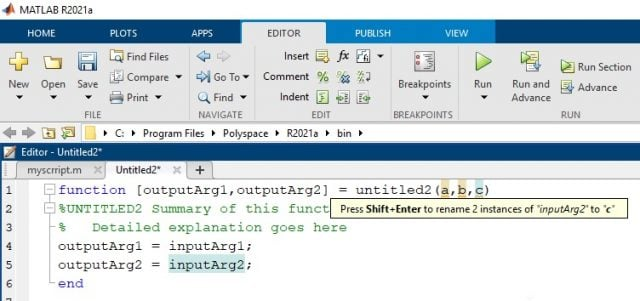You can enter any number of input arguments over here. So on the left side of this where it is untitled 3 is written. So this is basically the function name let me write here myfunction so this is the name of the function.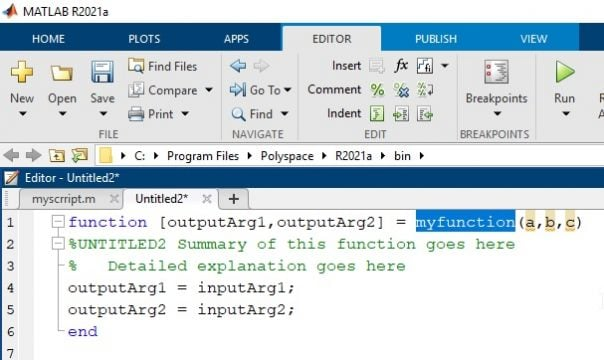Further left there are the output arguments. So in our case we have two output arguments the first one is sum and the second one is product.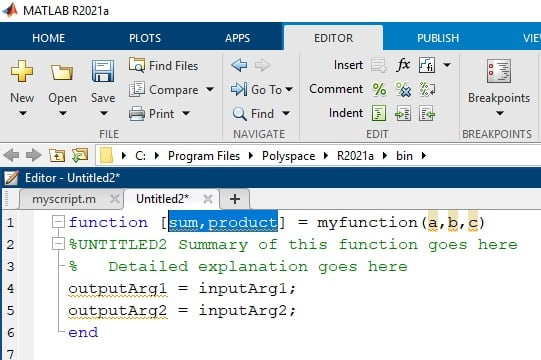Now this is the function de finition now let us write the functionality because it is function and the main difference between script and function is that function takes the input arguments externally. So we do not need to define a, b, and c inside function we will supply the values of a, b and c from outside and this function will return the sum and product in the form of output arguments. So here we will simply erase these three lines of the script.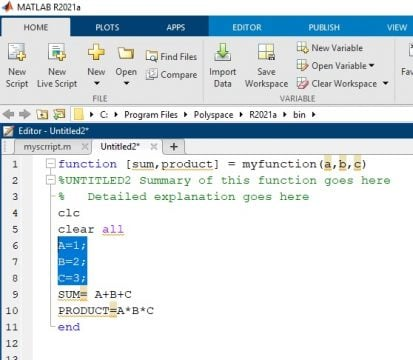So we will directly write:

SUM=A+B

PRODUCT=A*B*C

This is the completed function.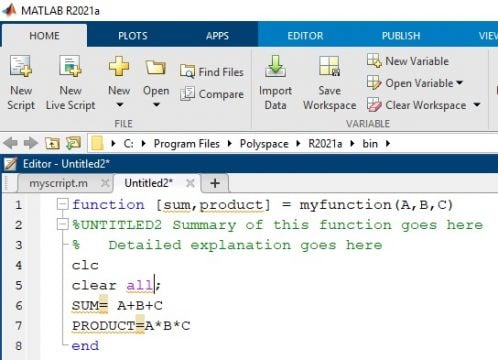Now before running you need to save again and one important point that you should keep in mind that you have to save this function by the same name that you have written in the first line in the description of this function.So here it is myfunction so MATLAB automatically suggests you and recommends you to save this file by the same name.You can see that myfunction is written.So you simply click save.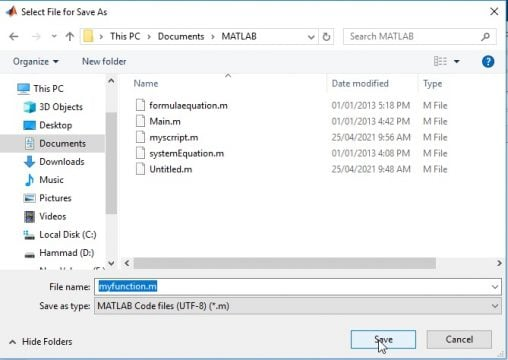So now to run this function you need to go either to a script or you need to goto the command window first of all. Let us go towards the command windowand now i write my function and inside the bracket I am writing the values of the arguments. Let’s say the values are 2, 3 4.

myfunction(2,3,4)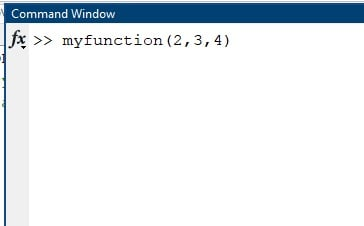So the value of a is 2 the value of b is 3 and the value of c is 4.Now i simply click enter so you see it has returned the two arguments.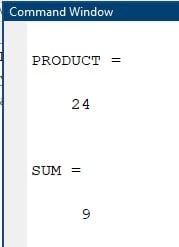So both the sum and product are returned in this particular case so this is the basic difference between script and function how you run it and how you provide the input and output

## Function example 1:

### calculating hypotenuse using Pythagoras theorem

Now let’s take a look at the first example that we are going to work in MATLAB. So we are going to use the Pythagoras theorem to calculate the hypotenuse of a right triangle. So according to the Pythagoras theorem:

Hyp2=base2+ perp2

When the length of the other two sides are known in this case our function is going to have two inputs and one output the input arguments are going to be the length of side a and the length of side B and the single output argument is going to be the hypotenuse C.

c2=a2+ b2

Which we are going to calculate based on the Pythagoras theorem.Let us move into MATLAB and implement this function the first thing I am going to do is start a new user-defined function file. So I will  click new and then function in a template pops up.  Our output argument is the hypotenuse that we will calculate with the Pythagoras theorem and I am going to use variable C for the hypotenuse. Our input arguments are the two sides of the right triangle that are going to be used to calculate the hypotenuse and that is a and b.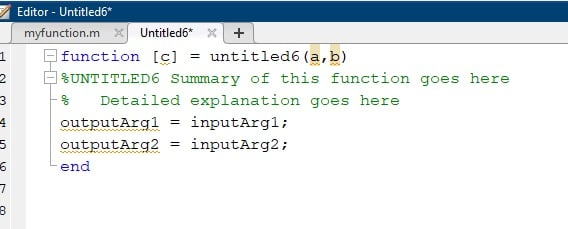Our function name I am going to call Pythagoras and when choosing a function name. Your main consideration is does this function name already exist in MATLAB to find out you can type exist then your potential function name that you would like to use and if you get a value of zero returned that indicates that this name is not already being used as a built-in function in MATLAB so you should be good to go to use that name. for example we are using the Pythagoras name for the function now to check it we will write in the command window:

Exist Pythagoras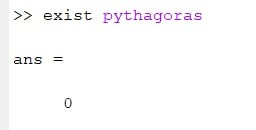As we have received 0 which shows that this function is not used before or it non exist in the predefined functions of the MATLAB.

Next we are going to go to our comment section and write a brief summary of the function. So in the function name a brief summary uses the Pythagoras theorem and a little bit more detail would be calculates the hypotenuse and that’s sufficient.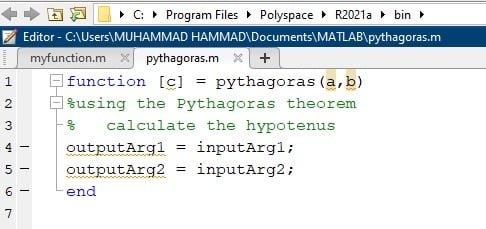Now we are going to calculate the hypotenuse based on the Pythagoras theorem. So our hypotenuse C is equal to the square root of a squared plus b squared.

c2=a2+ b2

In the MATLAB we will write this command as:

c = sqrt(a^2+b^2);

### Matlab code:

```function [c] = pythagoras(a,b)

%using the Pythagoras theorem

%   calculate the hypotenus

c = sqrt(a^2+b^2);

end```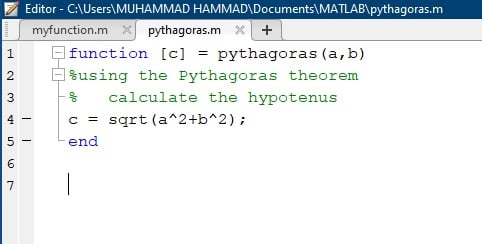So now what I want to do is I want to be able to calculate the hypotenuse based on two given side lengths so this is how we would call the function in the command window you would type in the function name and then you enter in your two inputs a and b. So let’s say side length of a is 3 and the length of side b is 4.

pythagoras(3,4)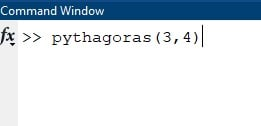When I press enter I will get a value of five which is what I’m expecting based on the Pythagorean theorem.